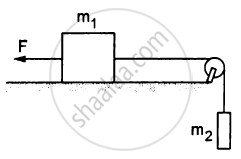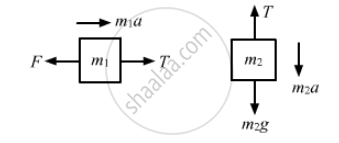# A Constant Force F = M2g/2 is Applied on the Block of Mass M1 as Shown in the Following Figure. the String and the Pulley Are Light and the Surface of the Table is Smooth. Find the Acceleration of M1. - Physics

Sum

A constant force F = m2g/2 is applied on the block of mass m1 as shown in the following figure. The string and the pulley are light and the surface of the table is smooth. Find the acceleration of m1.#### Solution

The free-body diagrams for both the blocks are shown below:From the free-body diagram of block of mass m1,
m1a = T − F    ...(i)

From the free-body diagram of block of mass m2,
m2a = m2g − T    ...(ii)

Adding both the equations, we get:
$a\left( m_1 + m_2 \right) = m_2 g - \frac{m_2 g}{2} \left......... [\text{ because F }= \frac{m_2 g}{2} \right]$
$\Rightarrow a = \frac{m_2 g}{2\left( m_1 + m_2 \right)}$
So, the acceleration of mass m1,
$a = \frac{m_2 g}{2\left( m_1 + m_2 \right)}, \text{ towards the right }.$

Concept: Newton’s Second Law of Motion
Is there an error in this question or solution?

#### APPEARS IN

HC Verma Class 11, Class 12 Concepts of Physics Vol. 1
Chapter 5 Newton's Laws of Motion
Q 26 | Page 81